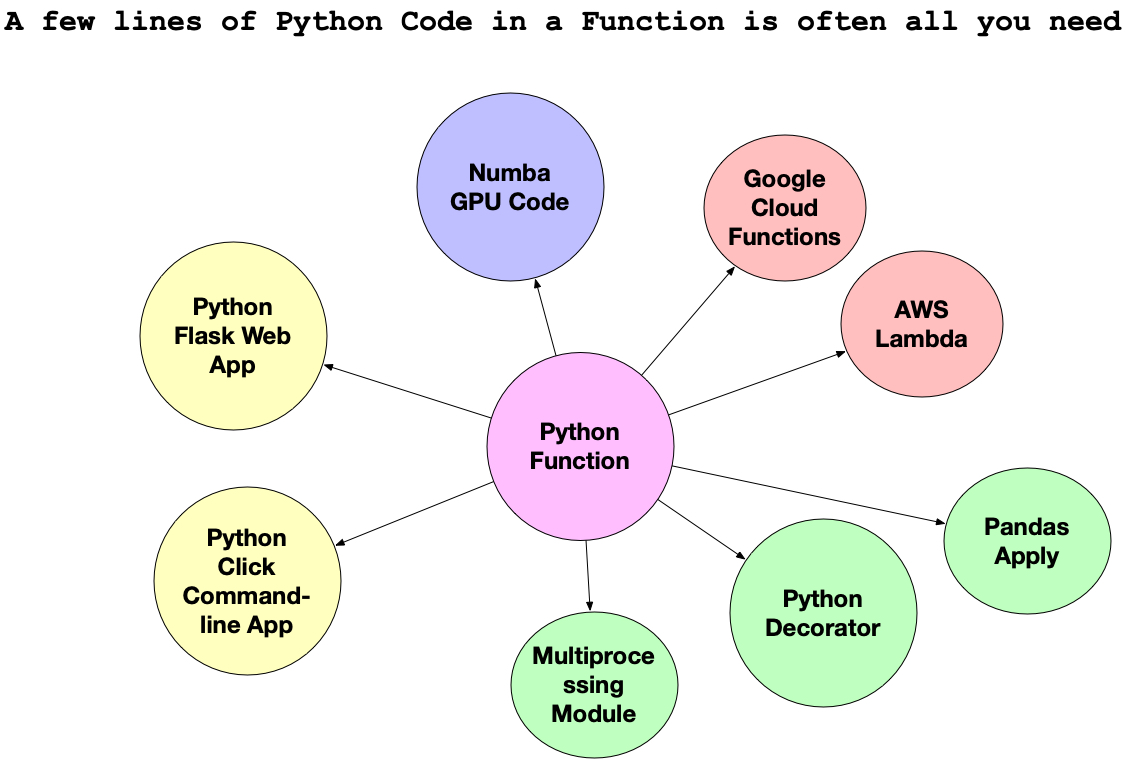Chapter03 Create Functions

If you find this content useful, consider buying this book:

• Amazon
• Purchase all books bundle
• Purchase from pragmatic ai labs
• Subscribe all content monthly
• If you enjoyed this book considering buying a copy

# Chapter 3: Learn to create functions #

The essential skill in the modern era of Python programming is the function. The reason many people don’t learn to program is they believe that mastery of other parts of Python is necessary. In the cloud and machine learning era, the function rules.

The Colab notebooks for this chapter can be found here.

## What can you do with a function? #

With just a few lines of code, many diverse and complex problems have solutions.Let’s take a look at a straightforward function that returns “Marco” if you pass in “Polo”.

``````def marco(name):
if name == "Marco":
return "Polo"
``````

This function takes in the `name` argument. If the name matches `Marco`, then it will return `Polo`. You can see this in action below. The function is called with the string `"Marco"` passed in.

``````marco("Marco")
``````
``````'Polo'
``````

Notice that if another name, in this case, `Bob` is passed it that it doesn’t return anything. The reason for this behavior is because, by default, a Python function will return `None`.

``````marco("Bob")
``````

As simple as this function is, it works in many different environments and targets. It can be “applied” to a Pandas DataFrame. It can run in the Cloud via AWS Lambda. Perhaps it could become a web service?

If you understand how a function works, you can program just about anything with the Python language! This concept is the secret that has kept many beginners from programming. It only takes a few lines of code to do incredible things. Not only that, but it is an excellent design to write small pieces of logic, even when building a more complex system.

Challenge: Write a simple function that takes a variable and returns something else if a phrase matches. For example something like this:

``````if dollars == 1000:
return "rich"
``````

Did it work? If not, let’s talk through some critical components of what makes a function work. It must have a few things to make it useful. A Python function uses the word `def` followed by some variable, in this case, `somename`. This syntax is what the name of the function is. It also has brackets `()` where the input goes and then is followed by `:`. Inside it needs to do something.

In the example above, it checked to see if the variable name was equal to `Marco`. Finally, it returns `someresult` which is a variable you create. A function doesn’t have to accept input, and it doesn’t need to return something explicitly, but most useful functions allow input and return some value.

``````def somename(somevar):
#some logic
return someresult
``````

### Function With No Input or Return #

What about a function that does even less? This example is a function that doesn’t take any input, and it doesn’t return anything. It just prints “Marco Polo”.

``````def simple_marco():
print("Marco Polo")
``````

If called it prints.

``````simple_marco()
``````
``````Marco Polo
``````

One way to check this is to assign the output of the function to a variable. You can then print that variable. In the following example, the variable `return_value` stores the output from the function, which is `None`, the default value of a function that has nothing to return.

``````return_value = simple_marco()
print(return_value)
``````
``````None
``````

### Function That Returns Without Accepting Input #

There are many cases where some logic performs in a function, but it doesn’t need to accept input. Here is an example where the two strings, `Marco` and `Polo` add together, then return.

``````def medium_marco():
statement = "Marco " + "Polo"
return statement
``````

The variable `return_medium_marco` shows that the `return` keyword is what tells the function to return `statement`. When the variable `return_medium_marco` prints, it returns `Marco Polo`.

``````return_medium_marco = medium_marco()
print(return_medium_marco)
``````
``````Marco Polo
``````

Challenge: Write a function with no input or return

### Function That That Accepts Input Without Return #

Let’s do another scenario. This example is a function that accepts input but only prints.

``````def input_marco(name):
if name == "Marco":
print("Polo")
else:
print("Nice try. No Cigar!")
``````

If `Marco` passes in, it will print `Polo`.

``````input_marco("Marco")
``````
``````Polo
``````

If another name passes in, it will run the other print statement.

``````input_marco("Bob")
``````
``````Nice try Bob. No Cigar!
``````

Notice that even though it prints, the function itself still returns `None`.

``````result = input_marco("Jenny")
print(result)
``````
``````Nice try Jenny. No Cigar!
None
``````

Challenge: Write a function with input and no return